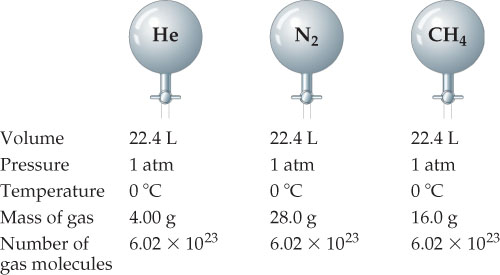# Problem: Avogadros hypothesis. At the same volume, pressure, and temperature, samples of different gases have the same number of molecules but different masses.How many moles of gas are in each vessel?

###### FREE Expert Solution

Recall that the molar volume of a gas at STP (273.15 K and 1 atm) is 22.4 L###### Problem DetailsAvogadros hypothesis. At the same volume, pressure, and temperature, samples of different gases have the same number of molecules but different masses.

How many moles of gas are in each vessel?